# Implementing SIFT in Python

EDIT: It seems like people are continuing to stumble across this. I just want to say that this article and the accompanying code (which I don’t maintain) have lots of mistakes and you should not consider this a super reliable resource. Keep reading if you want to pick up an implementation detail or two.

There’s a lot of content about SIFT online. There are a lot of good tutorials, but each seemed to be lacking something, whether that be details about the algorithm or the implementation. So when I decided I wanted to implement SIFT for myself, I found myself struggling to pull content from many, sometimes conflicting, sources. I have finally completed the task and have decided to share my process as well as some of the details other tutorials fail to mention.

# Introduction

These descriptors are useful for matching objects are patches between images. For example, consider creating a panorama. Assuming each image has some overlapping parts, you need some way to align them so we can stitch them together. If we have some points in each image that we know correspond, we can warp one of the images using a homography. SIFT helps with automatically finding not only corresponding points in each image, but points that are easy to match.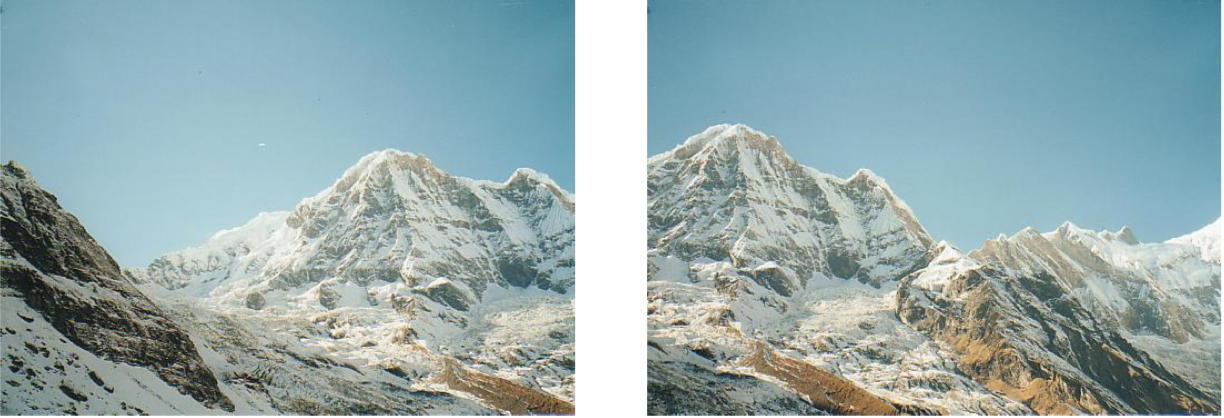Two images with an overlapping region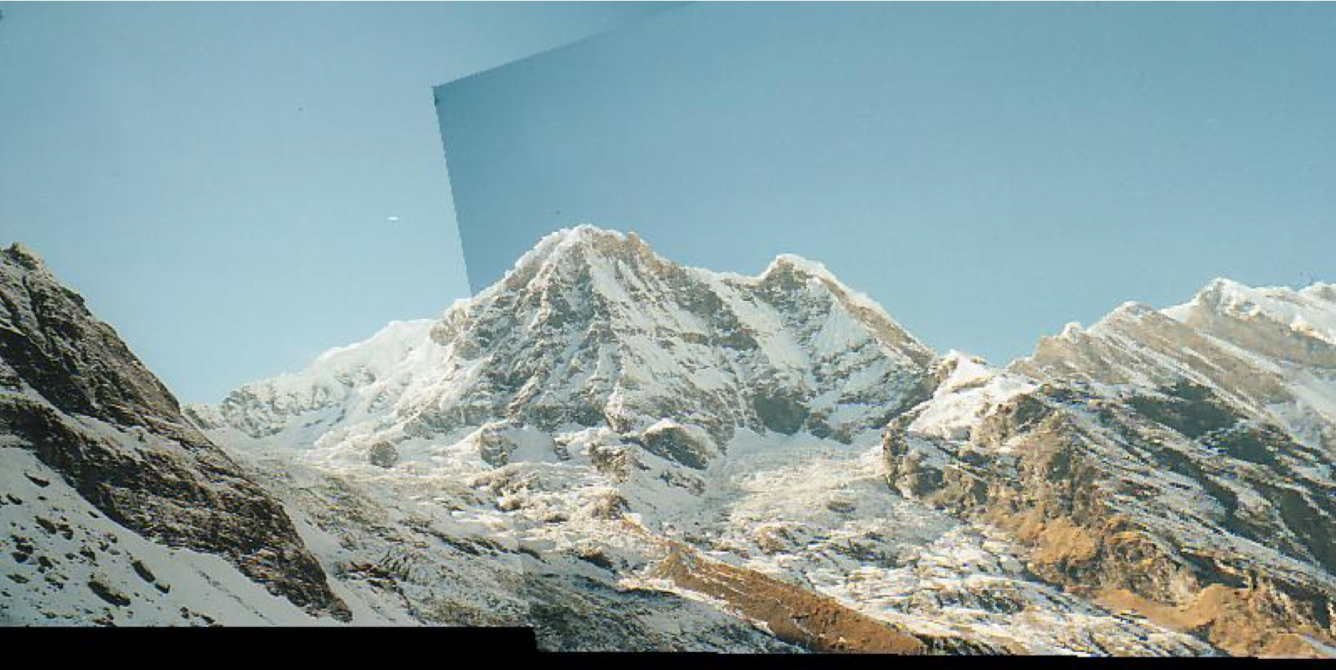Result after transforming one image using the homography from SIFT feature points

# Description of the Algorithm

1. Scale-Space Extrema Detection
2. Keypoint Localization
3. Orientation Assignment
4. Local Descriptor Creation

# Scale-Space Extrema Detection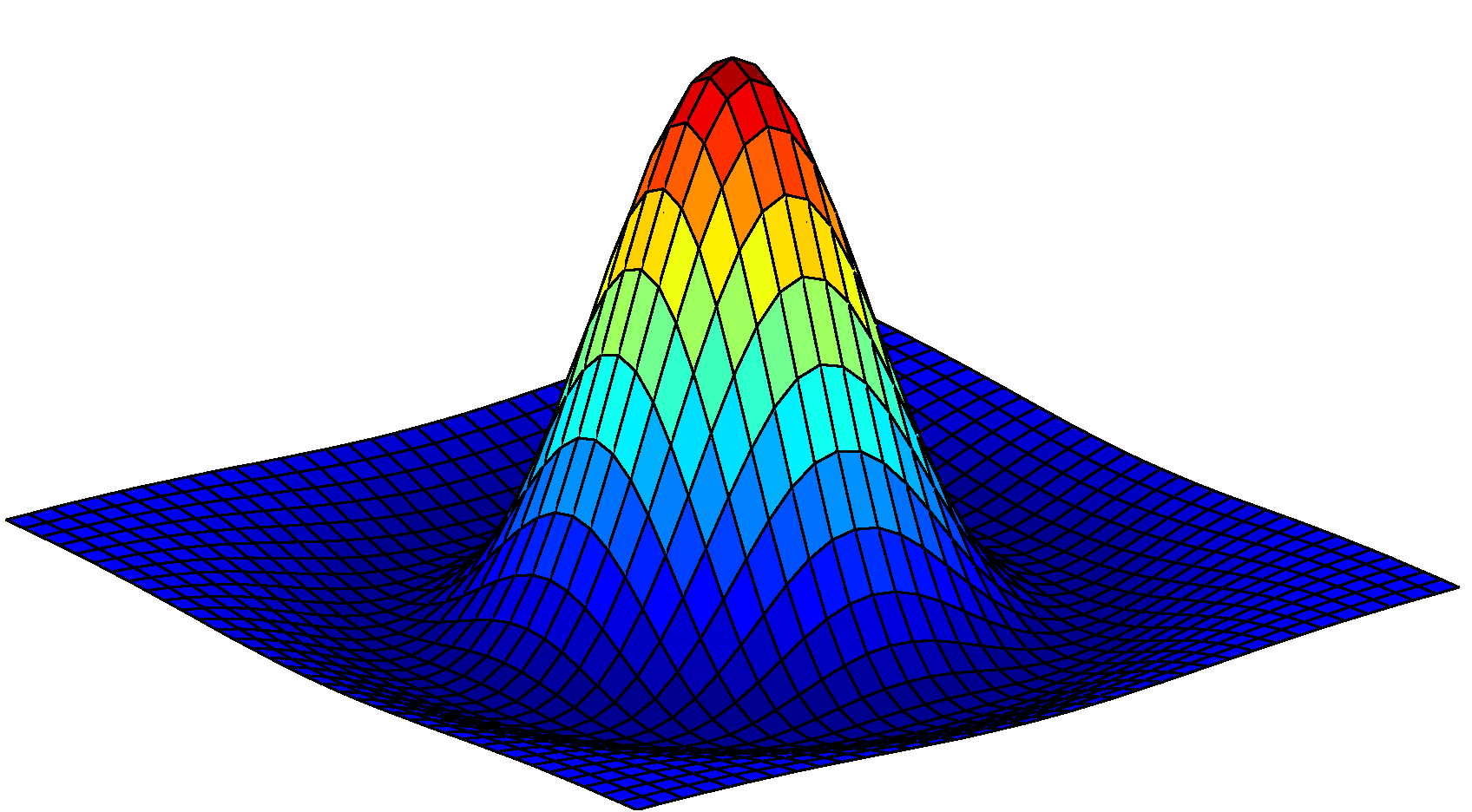Negative LoG

The LoG filter is highly peaked at the center while becoming slightly negative and then zero at a distance from the center characterized by the standard deviation, sigma, of the Gaussian.

Therefore, the LoG filter is most highly activated by a circle, or blob, with radius proportional to sigma. However, the Gaussian is normalized, i.e. if you integrate it over all space it sums to one. Therefore, with a higher sigma and therefore a wider Gaussian, the response of the LoG filter for that Gaussian will be lower than for a smaller sigma. Therefore, SIFT uses the scale-normalized LoG filter which is the regular LoG multiplied by the variance (sigma squared).

## DoG Approximation

It turns out that the difference of two Gaussians with similar variance yields a filter that approximates the scale-normalized LoG very well: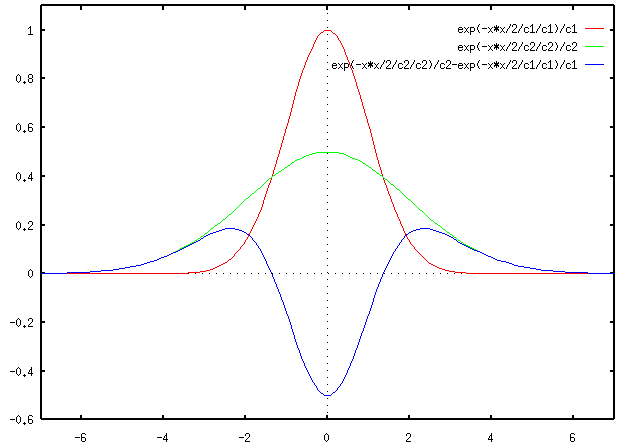The difference of two Gaussians approximates the Laplacian of a Gaussian

## DoG Pyramid

To create this octave, we first need to choose the number of images we want in each octave. This is denoted by s. Then the sigma for the Gaussian filter is chosen to be 2^(1/s). Since blur accumulates multiplicatively, when we blur the original image with this filter s times, the result will have blur = 2 * original blur.

One detail from the paper that I rarely see mentioned is that in each octave, you actually need to produce s+3 images (including the original image). This is because when adjacent levels are subtracted to obtain the approximated LoG octave, we will get one less image than in the Gaussian octave: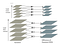DoG pyramid used for SIFT

Now we have s+2 images in the DoG octave. However, later when we look for extrema in the DoG, we will look for the min or max of a neighborhood specified by the current and adjacent levels. Obviously, this neighborhood cannot be obtained at the top or bottom level, so we have these two extra levels so that the neighborhood is defined over a full octave in the scale space. This will be more clear in the next section.

After we have generated the Gaussian octave, we downsample the top level by two and use that as the bottom level for a new octave. The original paper uses four octaves. These octaves make up what I call the Gaussian pyramid.

## Implementation

`def gaussian_filter(sigma):   size = 2*np.ceil(3*sigma)+1   x, y = np.mgrid[-size//2 + 1:size//2 + 1, -size//2 + 1:size//2 + 1]   g = np.exp(-((x**2 + y**2)/(2.0*sigma**2))) / (2*np.pi*sigma**2)  return g/g.sum()`

This assumes that numpy is already imported as np (as it always should be). I also put it in a file called `gaussian_filter.py`. Next, to generate a Gaussian octave, we simply need to select sigma (or k as it is called in the paper) and repeatedly convolve with this Gaussian filter:

`from scipy.ndimage.filters import convolve from gaussian_filter import gaussian_filter def generate_octave(init_level, s, sigma):   octave = [init_level]   k = 2**(1/s)   kernel = gaussian_filter(k * sigma)   for _in range(s+2):     next_level = convolve(octave[-1], kernel)     octave.append(next_level)   return octave`

Just as in the paper, I use an s of 5. One other thing to note is sigma parameter. This parameter is used to scale k so that the blur in each DoG octave goes from sigma -> 2*sigma. I use a value of 1.6 for sigma. Then to generate the whole Gaussian pyramid:

`def generate_gaussian_pyramid(im, num_octave, s, sigma):   pyr = []   for _ in range(num_octave):     octave = generate_octave(im, s, sigma)     pyr.append(octave)     im = octave[-3][::2, ::2]   return pyr`

Since we generate s+3 images per octave, we use the third to last image as the base for the next octave since that is the one with a blur of 2*sigma.

Now that we have the Gaussian pyramid, it is trivial to create the DoG pyramid:

`def generate_DoG_octave(gaussian_octave):   octave = []   for i in range(1, len(gaussian_octave)):       octave.append(gaussian_octave[i] — gaussian_octave[i-1])   return np.concatenate([o[:,:,np.newaxis] for o in octave], axis=2) def generate_DoG_pyramid(gaussian_pyramid):   pyr = []   for gaussian_octave in gaussian_pyramid:     pyr.append(generate_DoG_octave(gaussian_octave))   return pyr`

Now we have four DoG octaves, each with s+2 levels of blur. We can now move on to detecting extrema in the scale space.

## Extrema Detection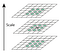Neighborhood for the initial scale-space extrema detection

This is why we generated s+2 levels in the DoG octave, since we cannot scan over the points in the top or bottom level, but we still want to get keypoints over a full octave of blur.

## Implementation

`def get_candidate_keypoints(D, w=16):   candidates = []   D[:,:,0] = 0   D[:,:,-1] = 0   for i in range(w//2+1, D.shape-w//2–1):     for j in range(w//2+1, D.shape-w//2–1):       for k in range(1, D.shape-1):         patch = D[i-1:i+2, j-1:j+2, k-1:k+2]         if np.argmax(patch) == 13 or np.argmin(patch) == 13:           candidates.append([i, j, k])   return candidates`

I have a few things to say about this code:

1. I set the top and bottom extra levels to 0 because I was initially only getting extrema in those levels. This is probably due an incorrect construction of the DoG octave, but it seems to work well enough.
2. Instead of scanning with a padding of one on each level, we have a padding of w/2 where w is the side length for the patches used when creating local descriptors. Without this, I was getting out of bounds errors.
3. Calling `argmax` or `argmin` without an axis parameter runs on the flattened array. Since our neighborhood is 3x3x3, or 27 elements long, when the extrema is our inspected pixel, the value of argmax or argmin will be 13.

These candidate keypoints yield many poor choices and/or noisy, so in the next section we will throw out bad ones as well refine good ones.

# Keypoint Localization

1. Compute the subpixel location of each keypoint
2. Throw out that keypoint if it’s scale-space value at the subpixel is below a threshold
3. Eliminate keypoints on edges using the Hessian around each subpixel keypoint

## Subpixel Localization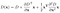Second-order Taylor expansion of the DoG octave

Here, x is the three-dimensional vector [x, y, sigma] corresponding to the pixel location of the candidate keypoint. Taking the derivative of this equation with respect to x and setting it equal to zero yields the subpixel offset for the keypoint: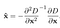Candidate keypoint offset (second-order)

This offset is added to the original keypoint location to achieve subpixel accuracy.

If you uncomfortable with this math, I would suggest reading the paper or some other sources on Taylor expansions because this post is already becoming a behemoth.

## Implementation

`def localize_keypoint(D, x, y, s):   dx = (D[y,x+1,s]-D[y,x-1,s])/2.   dy = (D[y+1,x,s]-D[y-1,x,s])/2.   ds = (D[y,x,s+1]-D[y,x,s-1])/2.   dxx = D[y,x+1,s]-2*D[y,x,s]+D[y,x-1,s]   dxy = ((D[y+1,x+1,s]-D[y+1,x-1,s]) — (D[y-1,x+1,s]-D[y-1,x-1,s]))/4.   dxs = ((D[y,x+1,s+1]-D[y,x-1,s+1]) — (D[y,x+1,s-1]-D[y,x-1,s-1]))/4.   dyy = D[y+1,x,s]-2*D[y,x,s]+D[y-1,x,s]   dys = ((D[y+1,x,s+1]-D[y-1,x,s+1]) — (D[y+1,x,s-1]-D[y-1,x,s-1]))/4.   dss = D[y,x,s+1]-2*D[y,x,s]+D[y,x,s-1]   J = np.array([dx, dy, ds])   HD = np.array([ [dxx, dxy, dxs], [dxy, dyy, dys], [dxs, dys, dss]])   offset = -LA.inv(HD).dot(J)  return offset, J, HD[:2,:2], x, y, s`

First, the first derivatives are computed for the Jacobian. Then, we compute the second derivatives for the Hessian. Thankfully, the Hessian is symmetric, so we only have to calculate six derivatives instead of nine. We return J and H because we will need them later.

As a side note, when a dimension of the offset is greater than 0.5, that means it is actually closer to another pixel-level keypoint. The paper says to redo this process with the candidate keypoint closer to the offset but I couldn’t get it to work.

Again, if you’re uncomfortable with multivariable calculus or don’t really understand what I’m saying, I suggest you read up on it before continuing.

## Discarding Low-Contrast Keypoints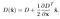Subpixel keypoint contrast

Which is the subpixel offset added to the pixel-level location. If the absolute value is below a threshold, we throw the point out. We do this because we want extrema that are, well, extreme.

## Eliminating Edge Responses

The Hessian that we return from `localize_keypoint` is of the form: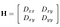The Hessian in scale space. Proportional to curvature

If you are familiar with the Harris detector, if the eigenvalues of H (say A and B) are both large, then the keypoint is most likely a corner. Conversely, if one is substantially larger, then it is most likely an edge.

Even though it is inexpensive to calculate the eigenvalues for this matrix, there is another way to do it involving taking the trace and determinant of H. We will forgo that discussing here but it is in the original paper.

## Implementation

`def find_keypoints_for_DoG_octave(D, R_th, t_c, w):   candidates = get_candidate_keypoints(D, w)  keypoints = []   for i, cand in enumerate(candidates):     y, x, s = cand, cand, cand     offset, J, H, x, y, s = localize_keypoint(D, x, y, s)     contrast = D[y,x,s] + .5*J.dot(offset)     if abs(contrast) < t_c: continue     w, v = LA.eig(H)     r = w/w     R = (r+1)**2 / r     if R > R_th: continue     kp = np.array([x, y, s]) + offset    keypoints.append(kp)  return np.array(keypoints)`

I use the values from the paper for `t_c` and `R_th`, namely 0.03 and (10+1)²/10 respectively. The motivation for `R_th` is more clear in the paper.

Now it is trivial to calculate the keypoints for the whole DoG pyramid:

`def get_keypoints(DoG_pyr, R_th, t_c, w):   kps = []   for D in DoG_pyr:     kps.append(find_keypoints_for_DoG_octave(D, R_th, t_c, w))   return kps`

# Orientation Assignment

We have translational invariance because we convolve our filters over the image. We also have scale invariance because of our use of the scale-normalized LoG filter. Now to impose rotational invariance, we assign the patch around each keypoint an orientation corresponding to its dominant gradient direction. This comes in handy later when we use the Histogram of Gradients descriptor to describe each patch.

To assign orientation, we take a patch around each keypoint thats size is proportional to the scale of that keypoint. We then create a histogram of the gradients for each pixel in that patch.

The histogram is created on angle (the gradient is specified in polar coordinates) and has 36 bins (each bin has a width of 10 degrees). When the magnitude and angle of the gradient at a pixel is calculated, the corresponding bin in our histogram grows by the gradient magnitude weighted by the Gaussian window.

Once we have our histogram, we assign that keypoint the orientation of the maximal histogram bin.

## Implementation

`def assign_orientation(kps, octave, num_bins=36):   new_kps = []   bin_width = 360//num_bins   for kp in kps:     cx, cy, s = int(kp), int(kp), int(kp)     s = np.clip(s, 0, octave.shape-1)     sigma = kp*1.5     w = int(2*np.ceil(sigma)+1)     kernel = gaussian_filter(sigma)     L = octave[…,s]    hist = np.zeros(num_bins, dtype=np.float32)     for oy in range(-w, w+1):       for ox in range(-w, w+1):         x, y = cx+ox, cy+oy         if x < 0 or x > octave.shape-1: continue         elif y < 0 or y > octave.shape-1: continue         m, theta = get_grad(L, x, y)         weight = kernel[oy+w, ox+w] * m         bin = quantize_orientation(theta, num_bins)         hist[bin] += weight     max_bin = np.argmax(hist)     new_kps.append([kp, kp, kp, fit_parabola(hist, max_bin, bin_width)])     max_val = np.max(hist)     for binno, val in enumerate(hist):       if binno == max_bin: continue       if .8 * max_val <= val:         new_kps.append([kp, kp, kp, fit_parabola(hist, binno, bin_width)])  return np.array(new_kps)`

The one thing I want to mention about this function is the last loop. The paper specifies that for any histogram bin that has a value within 80% of the maximum value to create a new keypoint at that location and scale but with the new orientation.

The paper also says that you should fit a parabola to the three histogram values closest to the maximum, which is done in the following function:

`def fit_parabola(hist, binno, bin_width):   centerval = binno*bin_width + bin_width/2.   if binno == len(hist)-1: rightval = 360 + bin_width/2.   else: rightval = (binno+1)*bin_width + bin_width/2.   if binno == 0: leftval = -bin_width/2.   else: leftval = (binno-1)*bin_width + bin_width/2.   A = np.array([     [centerval**2, centerval, 1],     [rightval**2, rightval, 1],     [leftval**2, leftval, 1]])   b = np.array([     hist[binno],     hist[(binno+1)%len(hist)],     hist[(binno-1)%len(hist)]])   x = LA.lstsq(A, b, rcond=None)   if x == 0: x = 1e-6   return -x/(2*x)`

We simply get the least squares solution where the center of the max histogram bin as well as its two adjacent bins are taken as the independent variable and the value at that histogram the dependent variable. Once the coefficients for the parabola have been found, just use -b/2a to get the refined orientation.

There are also a few functions not included in this code. First is `get_grads` , which gets the gradient in polar coordinates at a pixel in L:

`def cart_to_polar_grad(dx, dy):   m = np.sqrt(dx**2 + dy**2)   theta = (np.arctan2(dy, dx)+np.pi) * 180/np.pi   return m, theta def get_grad(L, x, y):   dy = L[min(L.shape-1, y+1),x] — L[max(0, y-1),x]   dx = L[y,min(L.shape-1, x+1)] — L[y,max(0, x-1)]   return cart_to_polar_grad(dx, dy)`

Then there is `quantize_orientation`, which simply converts the continuous angle of the gradient to a histogram bin:

`def quantize_orientation(theta, num_bins):   bin_width = 360//num_bins   return int(np.floor(theta)//bin_width)`

# Local Descriptor Creation

Specifically, a 16x16 patch is inspected around each keypoint. That patch is then split up into 16 4x4 subregions. The gradients (in polar coordinates) of each subregion are then binned into an 8-bin histogram. Finally, all of these histograms are concatenated into a 4x4x8=128 element long feature vector.

This final feature vector is then normalized, thresholded, and renormalized to try and ensure invariance to minor lighting changes.

## Implementation

`def get_local_descriptors(kps, octave, w=16, num_subregion=4, num_bin=8):   descs = []   bin_width = 360//num_bin  for kp in kps:     cx, cy, s = int(kp), int(kp), int(kp)     s = np.clip(s, 0, octave.shape-1)     kernel = gaussian_filter(w/6) # gaussian_filter multiplies sigma by 3     L = octave[…,s]     t, l = max(0, cy-w//2), max(0, cx-w//2)     b, r = min(L.shape, cy+w//2+1), min(L.shape, cx+w//2+1)     patch = L[t:b, l:r]     dx, dy = get_patch_grads(patch)     if dx.shape < w+1:       if t == 0: kernel = kernel[kernel.shape-dx.shape:]       else: kernel = kernel[:dx.shape]     if dx.shape < w+1:       if l == 0: kernel = kernel[kernel.shape-dx.shape:]       else: kernel = kernel[:dx.shape]     if dy.shape < w+1:       if t == 0: kernel = kernel[kernel.shape-dy.shape:]       else: kernel = kernel[:dy.shape]     if dy.shape < w+1:       if l == 0: kernel = kernel[kernel.shape-dy.shape:]       else: kernel = kernel[:dy.shape]     m, theta = cart_to_polar_grad(dx, dy)     dx, dy = dx*kernel, dy*kernel     subregion_w = w//num_subregion     featvec = np.zeros(num_bin * num_subregion**2, dtype=np.float32)     for i in range(0, subregion_w):       for j in range(0, subregion_w):         t, l = i*subregion_w, j*subregion_w         b, r = min(L.shape, (i+1)*subregion_w), min(L.shape, (j+1)*subregion_w)         hist = get_histogram_for_subregion(m[t:b, l:r].ravel(), theta[t:b, l:r].ravel(), num_bin, kp, bin_width, subregion_w)         featvec[i*subregion_w*num_bin + j*num_bin:i*subregion_w*num_bin + (j+1)*num_bin] = hist.flatten()     featvec /= max(1e-6, LA.norm(featvec))            featvec[featvec>0.2] = 0.2            featvec /= max(1e-6, LA.norm(featvec))        descs.append(featvec)  return np.array(descs)`

The checks on the shape of `dx` and `dy` in the middle are to avoid out of bounds errors I was getting earlier. If either/or are smaller than `w+1` then cut off the Gaussian window accordingly to avoid shape mismatches.

First, the polar gradients are computed for the whole patch. Then the histogram is created for each subregion. Once the histogram for each subregion has been concatenated, the entire feature vector is normalized, thresholded to be less than or equal to 0.2, and then renormalized.

I’m not 100% certain on the motivation behind the thresholding and renormalization but I believe it helps provide invariance to nonlinear lighting changes.

To calculate the gradients across the patch:

`def get_patch_grads(p):   r1 = np.zeros_like(p)   r1[-1] = p[-1]   r1[:-1] = p[1:]   r2 = np.zeros_like(p)   r2 = p   r2[1:] = p[:-1]   dy = r1-r2   r1[:,-1] = p[:,-1]   r1[:,:-1] = p[:,1:]   r2[:,0] = p[:,0]   r2[:,1:] = p[:,:-1]   dx = r1-r2   return dx, dy`

Just take the difference between the previous and current pixels. This is easier than doing the double-sided derivative as before. Then to create the histogram for each subregion:

`def get_histogram_for_subregion(m, theta, num_bin, reference_angle, bin_width, subregion_w):   hist = np.zeros(num_bin, dtype=np.float32)   c = subregion_w/2 - .5  for mag, angle in zip(m, theta):    angle = (angle-reference_angle) % 360            binno = quantize_orientation(angle, num_bin)            vote = mag             hist_interp_weight = 1 - abs(angle - (binno*bin_width + bin_width/2))/(bin_width/2)            vote *= max(hist_interp_weight, 1e-6)             gy, gx = np.unravel_index(i, (subregion_w, subregion_w))            x_interp_weight = max(1 - abs(gx - c)/c, 1e-6)                y_interp_weight = max(1 - abs(gy - c)/c, 1e-6)            vote *= x_interp_weight * y_interp_weight             hist[binno] += vote  hist /= max(1e-6, LA.norm(hist))   hist[hist>0.2] = 0.2   hist /= max(1e-6, LA.norm(hist))  return hist`

The code is fairly similar to the previous HoG code however we perform trilinear interpolation on each entry into the histogram. This interpolation is to provide robustness for when a gradient at a pixel either goes from one bin to the other or is at the edge of a subregion and goes into a different subregion.

Therefore, the weight of an entry is proportional to 1-(distance from the center of the bin) and well as 1-(distance from the center of the subregion) for both the x and y directions. The spatial (x and y) interpolation is the only thing I am not sure about because I could not find a reliable source on it.

# Putting it All Together

`class SIFT(object):   def __init__(self, im, s=3, num_octave=4, s0=1.3, sigma=1.6, r_th=10, t_c=0.03, w=16):     self.im = convolve(rgb2gray(im), gaussian_filter(s0))     self.s = s     self.sigma = sigma     self.num_octave = num_octave    self.t_c = t_c     self.R_th = (r_th+1)**2 / r_th     self.w = w   def get_features(self):     gaussian_pyr = generate_gaussian_pyramid(self.im, self.num_octave, self.s, self.sigma)     DoG_pyr = generate_DoG_pyramid(gaussian_pyr)     kp_pyr = get_keypoints(DoG_pyr, self.R_th, self.t_c, self.w)     feats = []     for i, DoG_octave in enumerate(DoG_pyr): kp_pyr[i] =       assign_orientation(kp_pyr[i], DoG_octave)       feats.append(get_local_descriptors(kp_pyr[i], DoG_octave))     self.kp_pyr = kp_pyr     self.feats = feats     return feats`

One final small detail is that I blur the initial image with a sigma of 1.3. I did not see this in the paper but I saw it in a few implementations.

# Conclusion

I’m aware that this is not a perfect implementation and nor does it implement every detail from the paper, but it empirically seems to work pretty well, whatever that means.

If you’re curious, here is a link to the complete repository (even though almost all the code is here): https://github.com/SamL98/PySIFT

Computer science enthusiast

## More from Sam Lerner

Computer science enthusiast